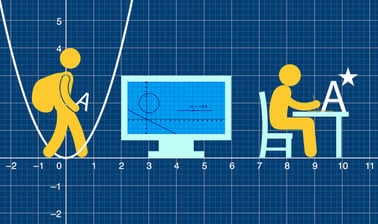# A-level Mathematics for Year 12 - Course 1: Algebraic Methods, Graphs and Applied Mathematics Methods

Develop your thinking skills, fluency and confidence to aim for an A* in A-level maths and prepare for undergraduate STEM degrees.### There is one session available:

35,634 already enrolled! After a course session ends, it will be archived.
Starts Sep 17
Estimated 7 weeks
2–4 hours per week
Self-paced
Free

This course by Imperial College London is designed to help you develop the skills you need to succeed in your A-level maths exams.

You will investigate key topic areas to gain a deeper understanding of the skills and techniques that you can apply throughout your A-level study. These skills include:

• Fluency – selecting and applying correct methods to answer with speed and efficiency
• Confidence – critically assessing mathematical methods and investigating ways to apply them
• Problem solving – analysing the ‘unfamiliar’ and identifying which skills and techniques you require to answer questions
• Constructing mathematical argument – using mathematical tools such as diagrams, graphs, logical deduction, mathematical symbols, mathematical language, construct mathematical argument and present precisely to others
• Deep reasoning – analysing and critiquing mathematical techniques, arguments, formulae and proofs to comprehend how they can be applied

Over seven modules, your initial skillset will be extended to give a clear understanding of how background knowledge underpins the A
-level course. You’ll also be encouraged to consider how what you know fits into the wider mathematical world.

### At a glance

• Institution: ImperialX
• Subject: Math
• Level: Intermediate
• Prerequisites:
None
• Language: English
• Video Transcript: English

# What you'll learn

Skip What you'll learn

• Improve fluency and accuracy when using laws of indices and surds in a variety of calculations
• Learn how to solve the types of inequalities you'll encounter at A-level and various ways to represent these
• Discover how to divide any polynomial by either a linear or quadratic polynomial
• Learn about the information found in different forms of the Cartesian equation of a circle and use these to solve coordinate geometry problems
• Investigate the main transformations of graphs; translation, enlargement and reflection, and use these transformations to sketch new graphs
• Understand the constant acceleration formulae through travel graphs illustration, speed, velocity, distance and displacement against time
• Explore statistical sampling methods and weigh up the advantages and disadvantages of each one
• Learn how to interpret data presented in a variety of forms including box plots, cumulative frequency curves, histograms and bar charts

# Syllabus

Skip Syllabus
Module 1  Indices and Surds
• Recognise and use the laws of indices for all rational exponents
• Use and manipulate surds, including rationalising the denominator
• Solve a variety of problems that include surds and indices
Module 2  Inequalities
• Solve linear and quadratic inequalities in a single variable and interpret these solutions graphically
• Express the solutions to linear and quadratic inequalities usingnumber lines and inequality notation, and using the terms ‘and’and ‘or’and set notation
• Represent linear and quadratic inequalities in two variables graphically, using standard A-level conventions
Module 3  The Factor Theorem & Algebraic Division
• Manipulate polynomials algebraically, using the factor theorem to write a polynomial as the product of linear factors or a combination of linear and quadratic factors
• Divide one polynomial by another of a lower order by equating coefficients
Module 4  Coordinate Geometry
• Solve problems using the coordinate geometry of the circle
• Complete the square to find the centre and radius of a circle from its equation
• Solve problems using the properties of the angle in a semicircle, the perpendicular from the centre to a chord, and a tangent from a poin
Module 5  Graphical Transformation and Curve Sketching
• Use curve sketching techniques based on the the shapes and symmetries of standard curves
• Identify key features of a curve from its equation and transform the equations of linear, quadratic, rational and trigonometrical curves using translations, rotations and stretches
• Use knowledge of the symmetry and asymptotes of standard curves to create sketches
Module 6  An Introduction to Mechanics
• Interpret and accurately use the term distance, speed, displacement, velocity, and acceleration
• Interpret graphs to do with speed against time, distance against time, velocity against time and acceleration against time, and solve problems involving motion in a straight line with constant acceleration
• Apply the formulae for constant acceleration to solve problems involving motion in a straight line
Module 7  An Introduction to Statistics
• Identify the ideas of a population and a sample and use simple sampling techniques to draw informal inferences about populations
• Apply critical thinking to issues of representative sampling
• Interpret histograms to draw informal inferences about univariate data
• Interpret scatter diagrams, regression lines and the ideas of correlation to draw informal inferences about bivariate data